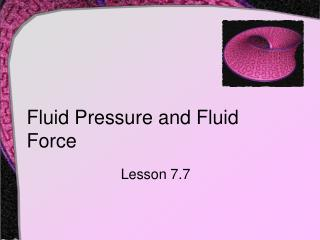DownloadDownload PresentationFluid Pressure and Fluid Force

Fluid Pressure and Fluid Force

Télécharger la présentationFluid Pressure and Fluid Force

- - - - - - - - - - - - - - - - - - - - - - - - - - - E N D - - - - - - - - - - - - - - - - - - - - - - - - - - -
Presentation Transcript

1. Fluid Pressure and Fluid Force Lesson 7.7

2. Fluid Pressure • Definition: The pressure on an object at depth h is • Where w is the weight-density of the liquid per unit of volume • Some example densitieswater 62.4 lbs/ft3mercury 849 lbs/ft3

3. Fluid Pressure • Pascal's Principle: pressure exerted by a fluid at depth h is transmitted equally in all directions • Fluid pressure given in terms of force per unit area

4. Fluid Force on Submerged Object • Consider a rectangular metal sheet measuring 2 x 4 feet that is submerged in 7 feet of water • Rememberso P = 62.4 x 7 = 436.8 • And F = P x Aso F = 436.8 x 2 x 4 = 3494.4 lbs

5. Fluid Pressure • Consider the force of fluidagainst the side surface of the container • Pressure at a point • Density x g x depth • Force for a horizontal slice • Density x g x depth x Area • Total force

6. 2.5 - y Fluid Pressure • The tank has cross sectionof a trapazoid • Filled to 2.5 ft with water • Water is 62.4 lbs/ft3 • Function of edge • Length of strip • Depth of strip • Integral (-4,2.5) (4,2.5) (2,0) (-2,0) y = 1.25x – 2.5x = 0.8y + 2 2 (0.8y + 2) 2.5 - y

7. Assignment A • Lesson 7.7 • Page 511 • Exercises 1-25 odd